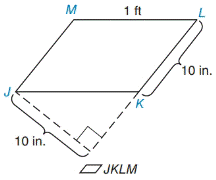Chapter 8.1, Problem 15EElementary Geometry For College St...

7th Edition
Alexander + 2 others
ISBN: 9781337614085

Solutions

Chapter
SectionElementary Geometry For College St...

7th Edition
Alexander + 2 others
ISBN: 9781337614085
Textbook Problem

In Exercises 9 to 18, find the areas of the figures shown or described.To determine

To find:

The area of the figure JKLM.

Explanation

1) Formula:

Area of the Parallelogram A=bh unit2.

Where, b is the base of the Parallelogram, and h is the height of the Parallelogram.

2) Calculation:

Given,

The given figure is a parallelogram JKLM with base b=10 in.,

Side s=1 ft=12 in.,

Let N be the point on the Parallelogram,

Where the LK is extended externally as LN which is perpendicular to JN that distance is 10 in

Still sussing out bartleby?

Check out a sample textbook solution.

See a sample solution

The Solution to Your Study Problems

Bartleby provides explanations to thousands of textbook problems written by our experts, many with advanced degrees!

Get Started

28. Write the first five terms of the sequence whose nth term is .

Mathematical Applications for the Management, Life, and Social Sciences

Finding Derivatives In Exercises 51-62, find f(x). f(x)=2x23x+1x

Calculus: An Applied Approach (MindTap Course List)

True or False: If R is a rectangular region in the plane, then

Study Guide for Stewart's Multivariable Calculus, 8th

limx2x2(x1)1x = _____. a) 4 b) 1 c) 0 d) does not exist

Study Guide for Stewart's Single Variable Calculus: Early Transcendentals, 8th